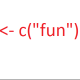# How to Add Frequencies as a Variable in SPSS

This post will cover how to add the number of times a certain response has been given for a variable (its frequency or count), and append this information to the data set as a new variable in SPSS. For example, say you have a list of names. Next to each name you’d like to add the number of times the name appears in the list. In SPSS, this information is easy to view with the “frequencies” dialogue, but how do you add it as a variable automatically?

Say you have this list of names in a variable called “name” in SPSS. See figure 1 for an example.

If you want to know how often each name appears in the list, simply use the “frequencies” command (Analyze > Descriptive Statistics > Frequencies) for the variable “name.” The output looks something like this.

Next to each name in the data set, we want a new variable with the number output by Frequencies. That is, we want every instance of “Kurt” to have “4” in this variable, every instance of “Paul” to have “2,” and all the others to have “1.”

In order to do this, SPSS needs an identifier variable. This is a variable that uniquely identifies each case in your data set. If your data set does not already have one (Qualtrics, for example, provides the identifier variable “ResponseID”), you must generate one. Go to Compute Variable (Transform > Compute Variable). Call the “Target Variable:” “ID” and in the “Numeric Expression:” block type “\$CASENUM”. This will add a variable called “ID” to the data set, the value of which will be the number of each case (rows are called cases in SPSS).

To add the frequencies as a variable, use the “Aggregate” function (Data > Aggregate). Bring the variable “name” over to the “Break Variable(s)” box. Next, bring the variable “ID” to the “Summaries of Variable(s):” box. With “ID_mean = MEAN(ID)” highlighted in the “Summaries of Variable(s)” box, click on the “Function…” button. From the right of the dialogue, select “Unweighted” under the heading “Number of cases.” Click “Continue.” The “Aggregated Variables” box now says “ID_nu = NU(ID).” Click “OK.” See figure 3.

1.Thank You! says: